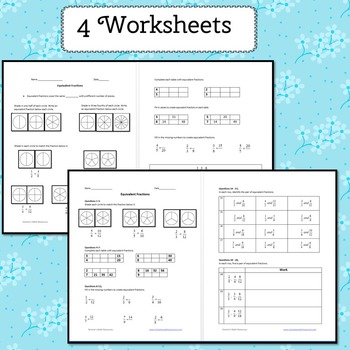# Equivalent Fractions Guided LessonSubject
Resource Type
File Type
Zip (2 MB|42 pages)
Standards
\$3.20
List Price:
\$4.00
You Save:
\$0.80
\$3.20
List Price:
\$4.00
You Save:
\$0.80
Also included in:
1. Equivalent Fractions guided Lesson and Adding and Subtracting Fractions guided Lesson. Each topic comes with guided and independent practice. You may also buy each product separately. Adding and Subtracting Fractions Guided Lesson Equivalent Fractions Guided lesson Included - Two Power Point fi
\$8.00
\$5.20
Save \$2.80
• Product Description
• Standards

Equivalent Fractions Guided lesson. The first half is guided practice which teaches the student about equivalent fractions. The second half is independent practice which allows the student to try one question at a time and then get instant feedback on the correct answer.

Included

- Power Point file with 37 interactive slides

-4 worksheets (2 guided and 2 independent)

Possible Uses

-Supplemental activity

-Centers or stations

-Introduce or review the topic

-Whole class instruction with a projector or any interactive white board

-Students may work independently on a computer

-Use the worksheets before, during, or after students view the PPT

-General Ed Class

-AIS, resource, math lab etc

CCSS

3.NF.3 Understand two fractions as equivalent if they are the same size, or the same point on a number line.

4.NF.1 Explain why a fraction a/b is equivalent to a fraction (n × a)/(n × b) by using visual fraction models, with attention to how the number and size of the parts differ even though the two fractions themselves are the same size.

*****************************************************************************

More Fractions

Multiply and Divide Fractions Scavenger Hunt

Adding and Subtracting Fractions PPT Lesson

Bingo

Multiplying And Dividing Fractions Flash Cards

Riddles

More Guided Lessons

Distance Between Points

Reflecting Points

Exponents

Combining Like Terms

Writing Expressions

Adding and Subtracting Fractions PPT Lesson

Coordinate Plane

*****************************************************************************

Follow me to be the first to know about sales, contests, and new products:

*****************************************************************************

Simone's Math Resources

Explain why a fraction 𝘢/𝘣 is equivalent to a fraction (𝘯 × 𝘢)/(𝘯 × 𝘣) by using visual fraction models, with attention to how the number and size of the parts differ even though the two fractions themselves are the same size. Use this principle to recognize and generate equivalent fractions.
Explain equivalence of fractions in special cases, and compare fractions by reasoning about their size.
Total Pages
42 pages